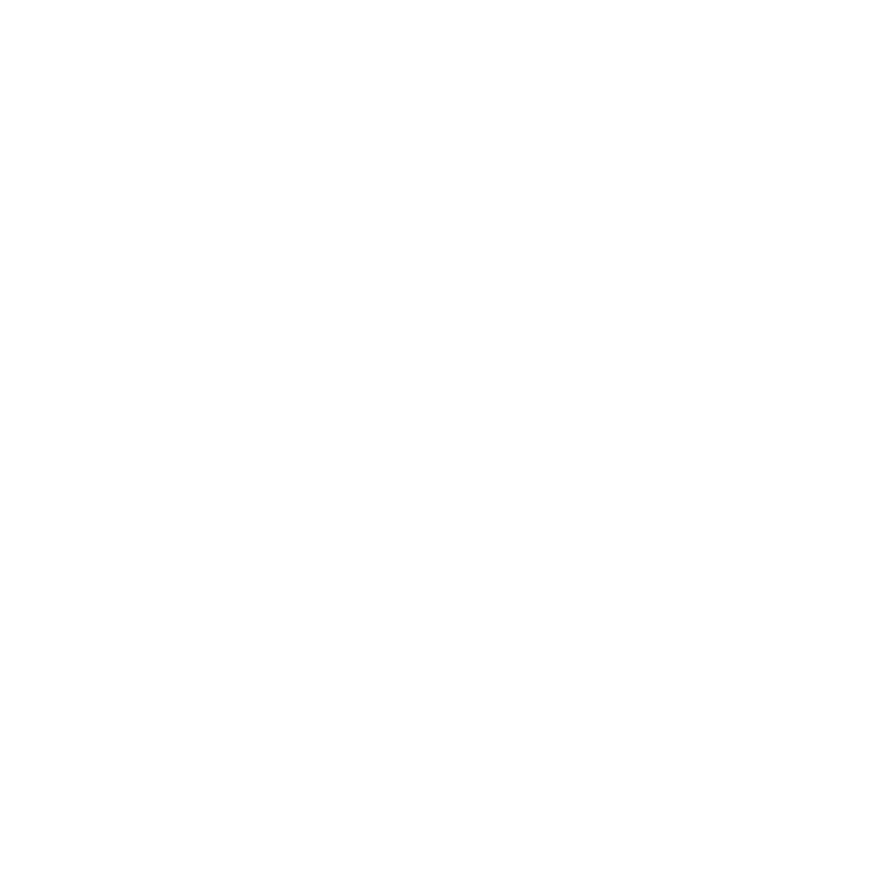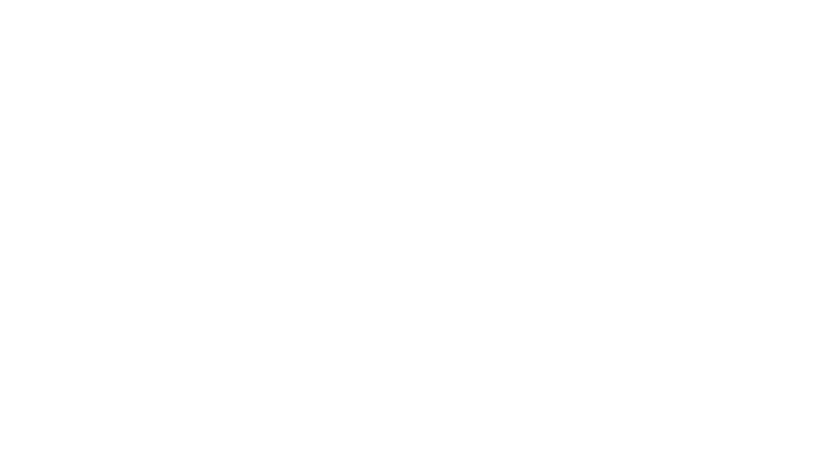Published on

# Creating a Circular Layout in SwiftUI

Authors
•Name
Parker Rex
@parkerrex

Hey there, today my friend Kayle is going to teach you how to create thsi view in SwiftUI. It requires a lot more than you'd think...Let's focus on the layout of the mini circles.

import SwiftUI

struct MiniCircle : View {
var title: String
var body: some View {
Circle()
.strokeBorder(Color.white,lineWidth: 2)
.background(Circle().foregroundColor(Color.accentColor))
.frame(width: 50, height: 50)
.overlay(Text(title).foregroundColor(.white))

}
}

struct ContentView: View {
@State private var count: Double = 1
var body: some View {
VStack {
Circle()
.foregroundColor(.init(hue: 0, saturation: 0.0, brightness: 0.9))

Slider(value: \$count, in: 1...16, step: 1).accentColor(.blue).padding(10.0)
Spacer(minLength: 10.0)
}
}

struct ContentView_Previews: PreviewProvider {
static var previews: some View {
ContentView()
}
}


First lets set up a GeometryReader in our base view to get the width of our view and set the dimensions of our background circle. We will need this metric later on when we are positioning our subviews.

GeometryReader { geometry in
Circle()
.foregroundColor(.init(hue: 0, saturation: 0.0, brightness: 0.9))
.frame(width: geometry.size.width, height: geometry.size.width, alignment:
.center)
.overlay(
CircularLayout(
radius: (geometry.size.width * 0.75) / 2,
count: Int(self.count))
.offset(x: geometry.size.width * 0.125, y: geometry.size.width * 0.125)
)
}


Next we will create our CirclularLayout with radius and count properties. The count is just for this simple demo and is the number of subviews to create.

struct CircularLayout : View {
var count: Int;

var body: some View {
}
}


Let’s add a ZStack to our CircularLayout to prevent other auto layout metrics from conflicting with the positions of our subviews.

struct CircularLayout : View {
var count: Int;

var body: some View {
ZStack {
}
}
}


Now we’ll add a ForEach to dynamically generate count number of views inside our ZStack.

struct CircularLayout : View {
var count: Int;

var body: some View {
ZStack {
ForEach((0...self.count-1), id: \.self) { index in
MiniCircle(title: "\(index)")
}
}
}


Before we start positioning the subviews, here’s a quick refresher on how the angle relates to the coordinates of a circle.Our X-Axis is radians and our Y-Axis is units. With our blue cos curve we see that for a 0 radian angle, we have a value of 1 and at PI we have a value of -1

Similarly, sin is shifted PI/2 radians so it is 0 at 0 and 1 at PI/2.

This lets us use (cos(angle), sin(angle)) as our coordinate for our positioned subview.

Cool, now let’s get out first view in the correct position. Start by moving it from (0,0) being top left to being in the center of our layout (self.radius, self.radius) This lets us just work from the Unit Circle for easy math.

struct CircularLayout : View {
var count: Int;

var body: some View {
ZStack {
ForEach((0...self.count-1), id: \.self) { index in
MiniCircle(title: "\(index)")
}
}
}


Next we will move our subview to the 3 o’clock position to start by adding cos(angle) * self.radius to the x and sin(angle) * radius to the y. We’ll workout what this angle is next.

struct CircularLayout : View {
var count: Int;

var body: some View {
ZStack {
ForEach((0...self.count-1), id: \.self) { index in
MiniCircle(title: "\(index)")
}
}
}


Remember that’s the form for a coordinate on a circle; adding or subtracting sin determines if we go clockwise or counterclockwise). Multiplying by the radius converts the Unit Circle to our circles dimensions.

To determine the angle: we know we want the views to be equidistant. So 2 views should give us an angle of PI to split the circle into 2. With 3 views we want 2PI/3 since there are 2PI radians in a circle. Here we can see the pattern is just 2PI/count to get an equidistant angle.

struct CircularLayout : View {
var count: Int;

var body: some View {
let angle = 2.0 / CGFloat(self.count) * .pi
return ZStack {
ForEach((0...self.count-1), id: \.self) { index in
MiniCircle(title: "\(index)")
}
}
}


Lastly we just want to multiply our angle by the index in the loop to apply a partial rotation around the circle for each subview.

struct CircularLayout : View {
var count: Int;

var body: some View {
let angle = 2.0 / CGFloat(self.count) * .pi
return ZStack {
ForEach((0...self.count-1), id: \.self) { index in
MiniCircle(title: "\(index)")
}
}
}


If you want to adjust where on the circle your first view is positioned, just add an offset to the cos and sin arguments. For example: cos(angle*index + PI/2) and sin(angle*index + PI/2) will start at 12 o’clock.

And that's it for how to build a CircularLayout.

I write a newsletter each tuesday. It's the best information I find every week, condensed into a 2 minute email that you receive 1x a week at 2pm et.

Join 2PM Tech Tuesdays

No Bs. Best Entrepreneurship, PM, Productivity and Investing links on the internet